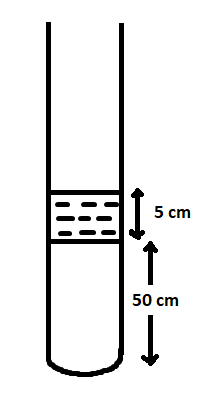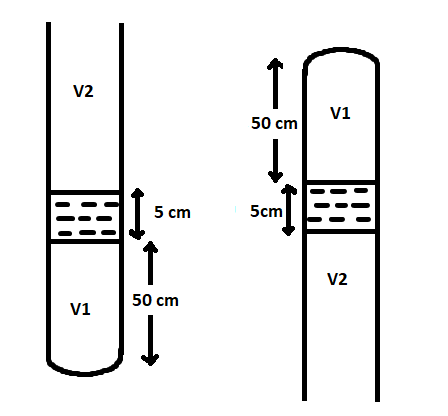# A vertical tube of length $100cm$ contains a mercury pallet of length $5cm$ as shown in the figure. Air is trapped between the mercury pallet and the base of the tube. The length $(cm)$ of the tube above the mercury pallet if the tube is inverted is nearly $(1atm = 75cm\, \, of\, \, Hg)$ . Assume air to be ideal gas and temperature to be constant.Verified
175.8k+ views
Hint: We have a tube and its length is given. There is a pallet of mercury at a given height and the air is trapped between the base of the tube and the mercury pallet. We have to find the length of the tube above the mercury pallet if the tube is inverted. The pressure of the atmosphere is given.

The air trapped between the base of the tube and the mercury pallet will experience pressure due to the mercury pallet and the pressure due to the atmosphere.Let $A$ be the cross-sectional area of the tube.
Let ${V_1}$ be the volume of the tube below the mercury pallet.
${V_1} = h \times A$
where $h$ is the height of volume ${V_1}$.
The height of mercury pallet is given as, $5cm = 0.05m$
Let ${P_{Hg}}$ be the pressure exerted by the mercury and ${P_{atm}}$ be the pressure exerted by the atmosphere.
When the tube is vertically inverted, the pressure on the trapped air be,
${P_{atm}} = {P_{Hg}} + {P_{air}}$

According to Pascal’s law, the pressure $P = h\rho g$
Where $P$stands for the pressure of the fluid, $h$stands for the height, $\rho$ stands for the density of the fluid, and $g$ stands for the acceleration due to gravity.
The height of the atmosphere above the mercury pallet is given as, $75cm = 0.75m$
The pressure of the atmosphere can be written as, ${P_{atm}} = 0.75\rho g$
The height of mercury pallet is given as, $5cm = 0.05m$
Therefore, the pressure of mercury can be written as, ${P_{Hg}} = 0.05\rho g$
Therefore we can write the equation
${P_{atm}} = {P_{Hg}} + {P_{air}}$ as,
$\Rightarrow 0.75\rho g = {P_{air}} + 0.05\rho g$
From this,
${P_{air}} = 0.75\rho g - 0.05\rho g = 0.70\rho g$

When the tube is kept upright,
The pressure experienced by the trapped air can be written as, ${P_{air}} = {P_{Hg}} + {P_{atm}}$
Now, we can write the total pressure on the trapped air as,
${P_{air}} = 0.05\rho g + 0.75\rho g = 0.80\rho g$
According to Boyle’s law,
${P_1}{V_1} = {P_2}{V_2}$
We know that Volume $V = h \times A$
where $h$is the height and $A$ stands for the area.
Now we can substitute the values of pressure and volume in Boyle’s law to get
$0.70\rho g \times h \times A = 0.80\rho g \times 50 \times A$
Cancelling common terms,
$0.70h = 0.80 \times 50$
From this, we get the height as,
$h = \dfrac{{0.80 \times 50}}{{0.70}} \approx 57.14cm$

Hence, $57.14cm$ is the answer.

Note: When the fluid is at rest, at any point in the same horizontal level, the fluid will have the same pressure. The pressure will be the same in all directions at any point in a liquid that is at rest. The pressure is always acting normal to the area whatever maybe the orientation of the area.